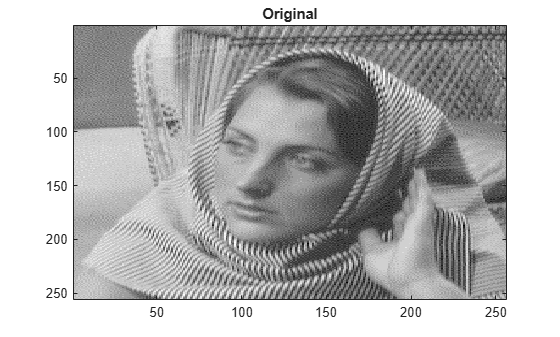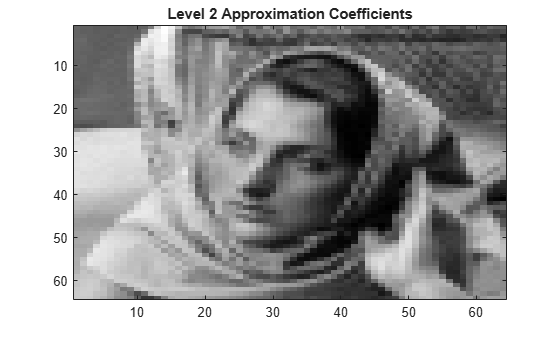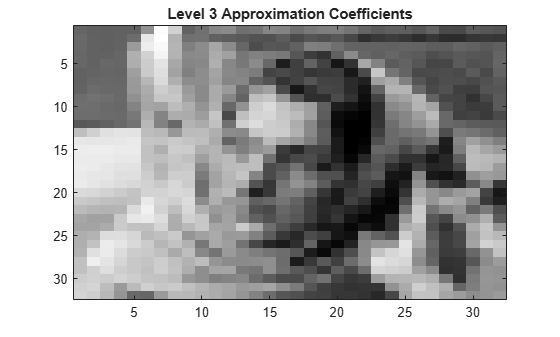Documentation

# appcoef2

2-D approximation coefficients

## Syntax

``A = appcoef2(C,S,wname)``
``A = appcoef2(C,S,LoR,HiR)``
``A = appcoef2(___,N)``

## Description

````A = appcoef2(C,S,wname)` returns the approximation coefficients at the coarsest scale using the wavelet decomposition structure [`C`,`S`] of a 2-D signal and the wavelet specified by `wname`. (See `wavedec2` for more information.)```
````A = appcoef2(C,S,LoR,HiR)` uses the lowpass reconstruction filter `LoR` and highpass reconstruction filter `HiR`. (See `wfilters` for more information.)```

example

````A = appcoef2(___,N)` returns the approximation coefficients at level `N`. If [`C`,`S`] is the `M`-level wavelet decomposition structure of a 2-D signal, then `0 ≤ N ≤ M`.```

## Examples

collapse all

This example shows how to reconstruct approximation coefficients from a multilevel wavelet decomposition of an image.

Set the DWT extension mode to zero-padding. Load and display an image.

```dwtmode('zpd','nodisp') load woman image(X) colormap(map) title('Original')````size(X)`
```ans = 1×2 256 256 ```

Perform a three-level wavelet decomposition of the image using the `db1` wavelet. Display the number of elements in the coefficients array `cfs`, and the contents of the bookkeeping matrix `inds`. Note that `cfs` has the same number of elements as `X`.

```wv = 'db1'; [cfs,inds] = wavedec2(X,3,wv); numel(X)```
```ans = 65536 ```
`numel(cfs)`
```ans = 65536 ```
`inds`
```inds = 5×2 32 32 32 32 64 64 128 128 256 256 ```

Extract and display the approximation coefficients at level 2.

```cfs2 = appcoef2(cfs,inds,wv,2); figure; imagesc(cfs2) colormap('gray') title('Level 2 Approximation Coefficients')````size(cfs2)`
```ans = 1×2 64 64 ```

Extract and display the approximation coefficients at level 3.

```cfs3 = appcoef2(cfs,inds,wv,3); figure; imagesc(cfs3) colormap('gray') title('Level 3 Approximation Coefficients')````size(cfs3)`
```ans = 1×2 32 32 ```

## Input Arguments

collapse all

Wavelet decomposition vector of a 2-D signal, specified as a real-valued vector. `C` is the output of `wavedec2`. The bookkeeping matrix `S` contains the dimensions of the coefficients by level.

Example: `[C,S] = wavedec2(randn(256,256),4,'db4')` returns the 4-level wavelet decomposition of a matrix.

Data Types: `double`

Bookkeeping matrix of the wavelet decomposition of a 2-D signal, specified as a matrix of positive integers. The bookkeeping matrix is used to parse the coefficients in the wavelet decomposition vector `C` by level.

Example: `[C,S] = wavedec2(randn(256,256),4,'db4')` returns the 4-level wavelet decomposition of a matrix.

Data Types: `double`

Wavelet used to generate the wavelet decomposition of a 2-D signal, specified as a character vector or string scalar. The wavelet is from one of the following wavelet families: Daubechies, Coiflets, Symlets, Fejér-Korovkin, Discrete Meyer, Biorthogonal, and Reverse Biorthogonal. See `wavemngr` for the wavelets available in each family.

Example: `'db4'`

Wavelet lowpass reconstruction filter, specified as an even-length real-valued vector. `LoR` must be the same length as `HiR`. `LoR` must be the lowpass reconstruction filter associated with the wavelet used to create the wavelet decomposition structure [`C`,`S`]. (See `wfilters` for more information.)

Wavelet highpass reconstruction filter, specified as an even-length real-valued vector. `HiR` must be the same length as `LoR`. `HiR` must be the highpass reconstruction filter associated with the wavelet used to create the wavelet decomposition structure [`C`,`S`]. (See `wfilters` for more information.)

Approximation coefficients level, specified as a positive integer. If [`C`,`S`] is the `M`-level wavelet decomposition structure of a 2-D signal, then `0 ≤ N ≤ M`.

## Output Arguments

collapse all

Approximation coefficients at level `N`, returned as a real-valued matrix or 3-D real-valued array. If `C` and `S` are obtained from an indexed image analysis or a truecolor image analysis, `A` is an `m`-by-`n` matrix or an `m`-by-`n`-by-3 array, respectively.

For more information on image formats, see `image` and `imfinfo`.

## Algorithms

The input vector `C` and bookkeeping matrix `S` contain all the information about the 2-D signal decomposition.

Let `NMAX = size(S,1)-2`; then ```C = [A(NMAX) H(NMAX) V(NMAX) D(NMAX) … H(1) V(1) D(1)]``` where `A`, `H`, `V`, and `D` are vectors. If `N = NMAX`, then a simple extraction is done; otherwise, `appcoef2` computes iteratively the approximation coefficients using the inverse wavelet transform.

## See Also

#### Introduced before R2006a

##### SupportGet trial now